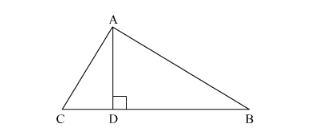# In a ∆ABC, perpendicular AD from A and BC meets BC at D. If BD = 8 cm, DC = 2 cm and AD = 4 cm, then

Question:

In a ∆ABC, perpendicular AD from A and BC meets BC at D. If BD = 8 cm, DC = 2 cm and AD = 4 cm, then

(a) ∆ABC is isosceles
(b) ∆ABC is equilateral
(c) AC = 2AB
(d) ∆ABC is right-angled at A

Solution:

Given: $\ln \triangle \mathrm{ABC}, \mathrm{AD} \perp \mathrm{BC}, \mathrm{BD}=8 \mathrm{~cm}, \mathrm{DC}=2 \mathrm{~cm}$ and $\mathrm{AD}=4 \mathrm{~cm}$.$\mathrm{AC}^{2}=\mathrm{AD}^{2}+\mathrm{DC}^{2}$

$\mathrm{AC}^{2}=4^{2}+2^{2}$

$\mathrm{AC}^{2}=20$......(1)

$\mathrm{AB}^{2}=\mathrm{AD}^{2}+\mathrm{BD}^{2}$

$\mathrm{AB}^{2}=4^{2}+8^{2}$

$\mathrm{AB}^{2}=80$.....(2)

Now, In ΔABC

BC2=CD+DB2=2+82=102=100

and

AB2+CA2=80+20=100

$\therefore \mathrm{AB} 2+\mathrm{CA} 2=\mathrm{BC} 2$

Hence, triangle ABC is right angled at A.

We got the result as (d)